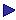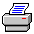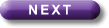Tutorial Contents Tutorial Seven: Designators - Designators, the tableau rules and individual constants - Using Russell's theory of descriptions - An oddity More Tutorials OneTwoThreeFourFiveSixAn oddity

When one is doing a tableau and one has "xFx in a branch, one can add Fa only if "a" appears in the branch. The reason is that "a" can be used only if it is safe to suppose, for the purposes of considering that branch, that there is something for it to refer to. The easiest way for it to be safe is if "a" already occurs in the branch. (Actually it would also be perfectly safe as long as some designator, say "b", occurred in the branch; but the rules don't need to be that generous.)

Nonetheless ""xFx; so Fa" is valid. ("xFx|-Fa is a correct sequent.)

Proof:

 "xFx ¬Fa   Fa

The answer is that, as we have seen, one cannot translate a designator in an argument (or in any set of sentences) with "a" (or any individual constant) unless one can take it that the designator refers to something (in one's domain of quantification). So, given the assumption that an argument has been correctly translated as ""xFx; so Fa", one can take it for granted that "a" refers to something. But, if one can take it that there is such a thing as a, one can see that "Fa" must be true if ""xFx" is.

Notice, however, that one cannot prove "xFx|-\$xFx. And, given that we cannot assume that we are not dealing with an empty domain, this is as it should be. Whereas the very use of "a", assuming it to be a correct use, implies the existence of something in the domain, the correct use of "\$x" does not. (e.g. "¬\$xFx" does not imply the existence of something, but "¬Fa" does.)

N.B. incidentally that this has the curious consequence that "xFx|-Fa is correct, and so is Fa|-\$xFx; but "xFx|-\$xFx is not.

A related point

Everything is brown; so the cow is brown.

If one thought that one could take it for granted that there was such a thing as the cow, one could translate the argument as,

"xBx; so Ba. (Valid)

If one did not think one could take this for granted one could use Russell's method and translate it as,

"xBx; so \$x[[CxÙ"y[Cy®y=x]]ÙBx] (Invalid)

If one thought one could take it that there was such a thing as the cow and one wished to use Russell's method, one could add an additional premise to produce the valid argument,

\$x[CxÙ"y[Cy®y=x]], "xBx; so \$x[[CxÙ"y[Cy®y=x]]ÙBx]Print this page